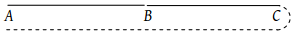A point starts moving in a straight line with a certain acceleration. At a time t after beginning of motion the acceleration suddenly becomes retardation of the same value. The time in which the point returns to the initial point is

(1) $\sqrt{2t}$

(2) $\left(2+\sqrt{2}\right)\text{\hspace{0.17em}}t$

(3) $\frac{t}{\sqrt{2}}$

(4) Cannot be predicted unless acceleration is given

Concept Videos :-

#16-Acceleration
#17-Solved-Examples-9
#18 Solved Examples 10
#19-Solved-Examples-11

Concept Questions :-

Acceleration

(2) In this problem point starts moving with uniform acceleration a and after time t (Position B) the direction of acceleration get reversed i.e. the retardation of same value works on the point. Due to this velocity of points goes on decreasing and at position C its velocity becomes zero. Now the direction of motion of point reversed and it moves from C to A under the effect of acceleration a.

We have to calculate the total time in this motion. Starting velocity at position A is equal to zero.

Velocity at position B v = at [As u = 0]Distance between A and B, ${S}_{AB}=\frac{1}{2}a{t}^{2}$

As same amount of retardation works on a point and it comes to rest therefore ${S}_{BC}={S}_{AB}=\frac{1}{2}a\text{\hspace{0.17em}}{t}^{2}$

${S}_{AC}={S}_{AB}+{S}_{BC}=a\text{\hspace{0.17em}}{t}^{2}$ and time required to cover this distance is also equal to t.

∴ Total time taken for motion between A and C = 2t

Now for the return journey from C to A $\left({S}_{AC}=a\text{\hspace{0.17em}}{t}^{2}\right)$

${S}_{AC}=u\text{\hspace{0.17em}}t+\frac{1}{2}a{t}^{2}$$a\text{\hspace{0.17em}}{t}^{2}=0+\frac{1}{2}a\text{\hspace{0.17em}}{t}_{1}^{2}$${t}_{1}=\sqrt{2}\text{\hspace{0.17em}}t$

Hence total time in which point returns to initial point

$T=2t+\sqrt{2}\text{\hspace{0.17em}}t=\left(2+\sqrt{2}\text{\hspace{0.17em}}\right)t$

Difficulty Level:

• 16%
• 57%
• 12%
• 17%